•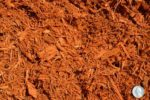•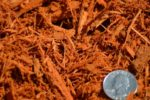•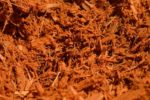•# Honey Gold

Looking for a brightening update for your yard? Look no further than our premium honey gold mulch. With a golden color, your yard or workspace is sure to shine this summer. Our mulch is colored with environmentally safe dyes and is great for weed suppression.

• Shredded for optimal look and feel
• Natural looking golden color
• Use one of our colored mulches to spice up your project.
• Effective in repelling insects and fungus
If you need help finding out your total cubic yardage use one of our calculators below!
Aerial Map Calculator
Area Totals
Current Area
0 ft2
Total Area
0 ft2
Total Measurement: 0 cubic yards
Total Price: \$0
Square Footage Calculator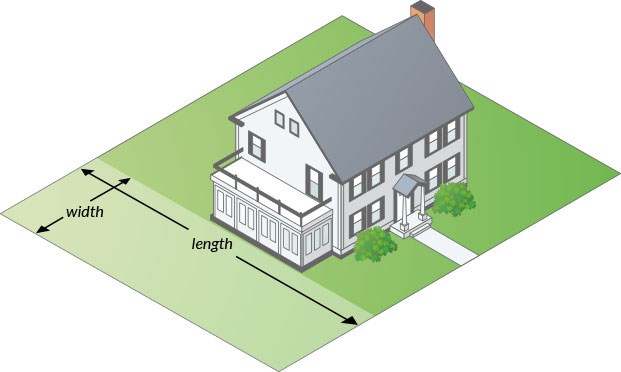#### How to measure your area for mulch

• Area 1
• Area 1
• Area 1
Total Measurement: 0 cubic yards
Total Price: \$0

### How To Measure

#### Square or Rectangle

Measure the Length & Width and multiply.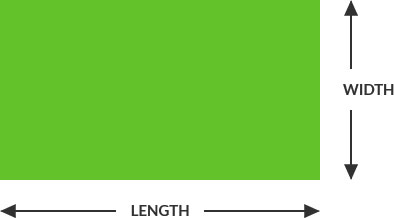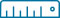Length x Width = Area
(12’ x 24’ = 288 ft2)

#### Right Triangle

Multiply the two sides that contact the right angle and divide by two.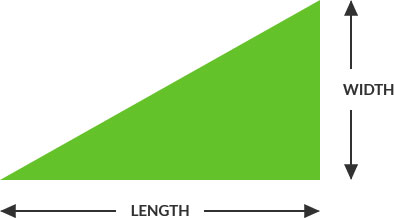Length x Width / 2 = Area
(12’ x 24’ = 288 ÷ 2 = 144 ft2)

#### Circular

Multiply the radius with the radius then multiply by 3.14. Radius is a straight line from the center of a circle to the outside of the circle.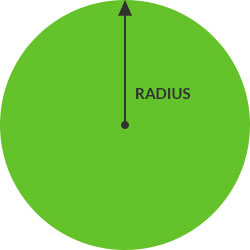##### Full Circle:(6’ x 6’ = 36 x 3.14 = 113 ft2)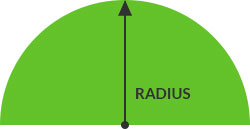##### Half Circle:Divide full circle radius by 2
(6’ x 6’ = 36 x 3.14 = 113 / 2 = 56.5 ft2)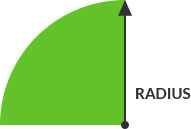##### Quarter Circle:Divide full circle radius by 4
(6’ x 6’ = 36 x 3.14 = 113 / 4 = 28.25 ft2)